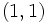# GAP:IrrepDegreeGroupingFull

## Definition

### Function type

This is a coded (not in-built) function in GAP to group together all groups of a given order based on their degrees of irreducible representations.

### Behavior

The function is invoked as:

IrrepDegreeGroupingFull(n)

where$n$ is a natural number. The output is a list of pairs of lists, where each pair is as follows:

• The first member of the pair is itself a list of pairs describing a collection of degrees of irreducible representations over$\mathbb{C}$: the first member of each pair is a degree and the second member is the number of irreducible representations of that degree.
• The second member of the pair is the list of second parts of GAP IDs of groups of order$n$ having those degrees of irreducible representations.

## Code

```IrrepDegreeGroupingFull := function(n)
local L,M,m,N;
L := List(AllSmallGroups(n),CharacterDegrees);
M := SortedList(Set(L));
m := Length(L);
N := List(M,i -> [i,Filtered([1..m],j->L[j]=i)]);
return(SortedList(N));
end;;```

## Examples of usage$n$ Function call Output Interpretation
1 IrrepDegreeGroupingFull(1) [ [ [ [ 1, 1 ] ], [ 1 ] ] ] The group with GAP ID$(1,1)$ has 1 irreducible representation of degree 1.
8 IrrepDegreeGroupingFull(8) [ [ [ [ 1, 4 ], [ 2, 1 ] ], [ 3, 4 ] ], [ [ [ 1, 8 ] ], [ 1, 2, 5 ] ] ] The groups with GAP IDs$(8,3)$ and$(8,4)$ both have four irreducible representations of degree 1 and one irreducible representation of degree 2. The groups with GAP IDs$(8,1)$,$(8,2)$, and$(8,5)$ each have 8 irreducible representations of degree 1.